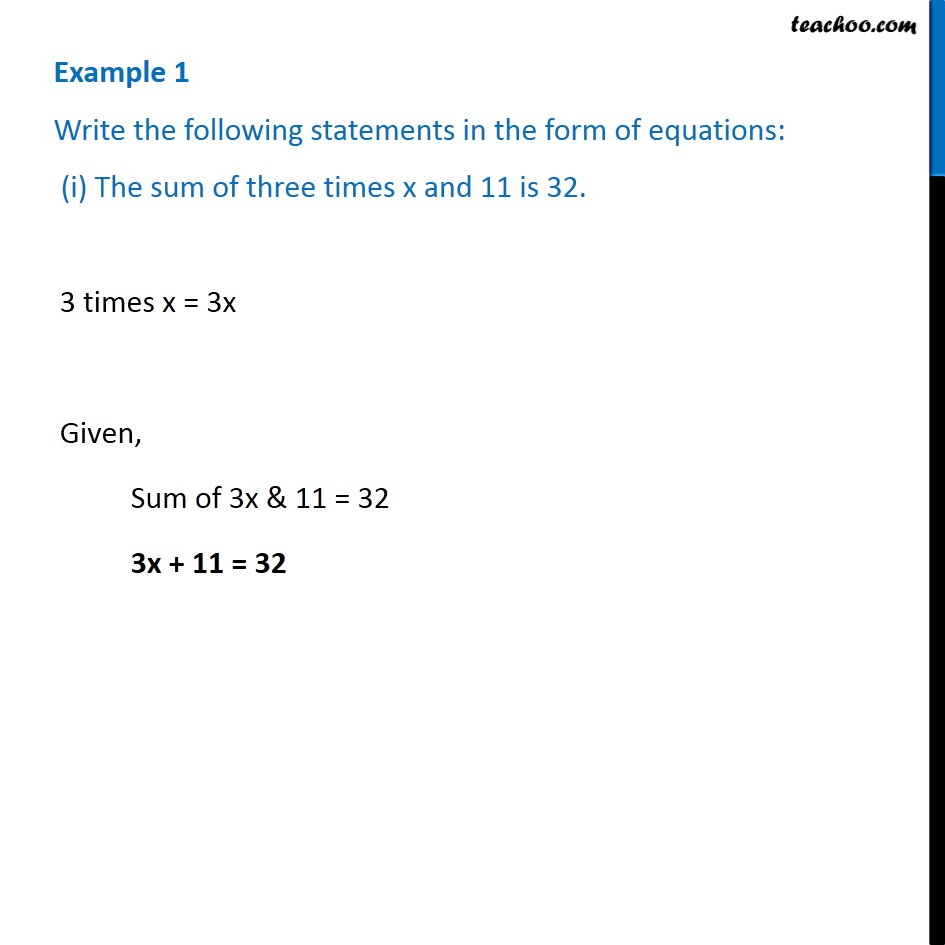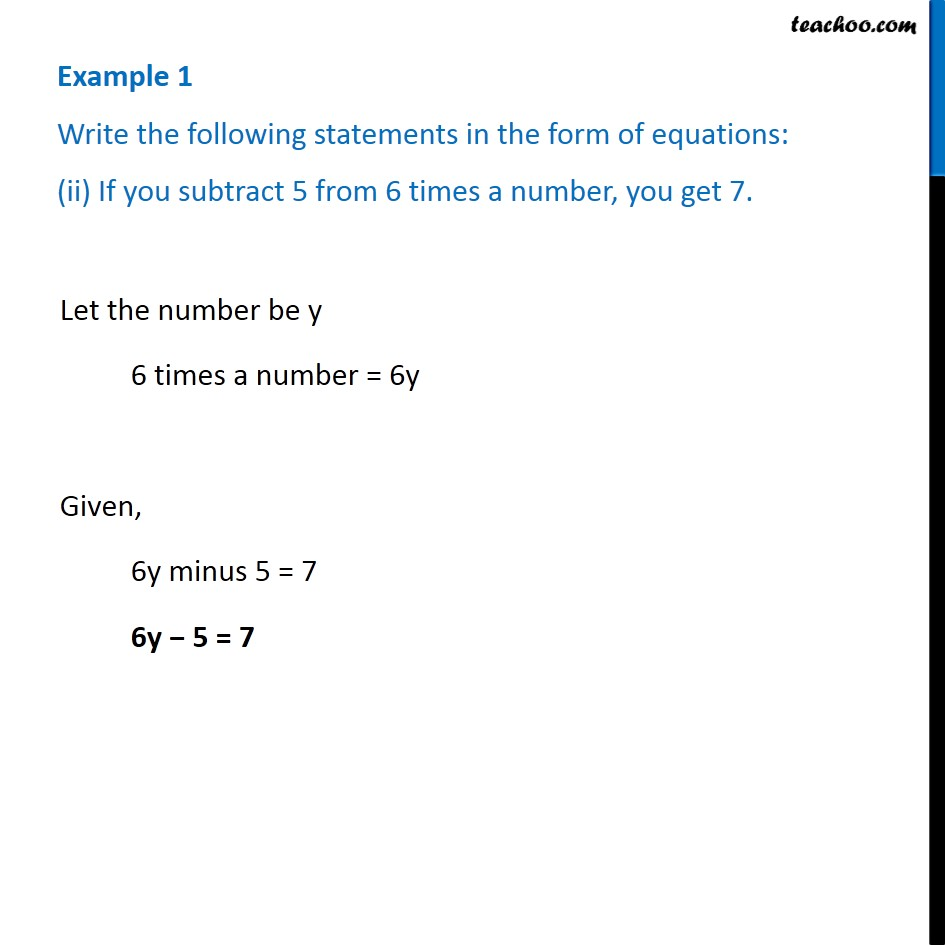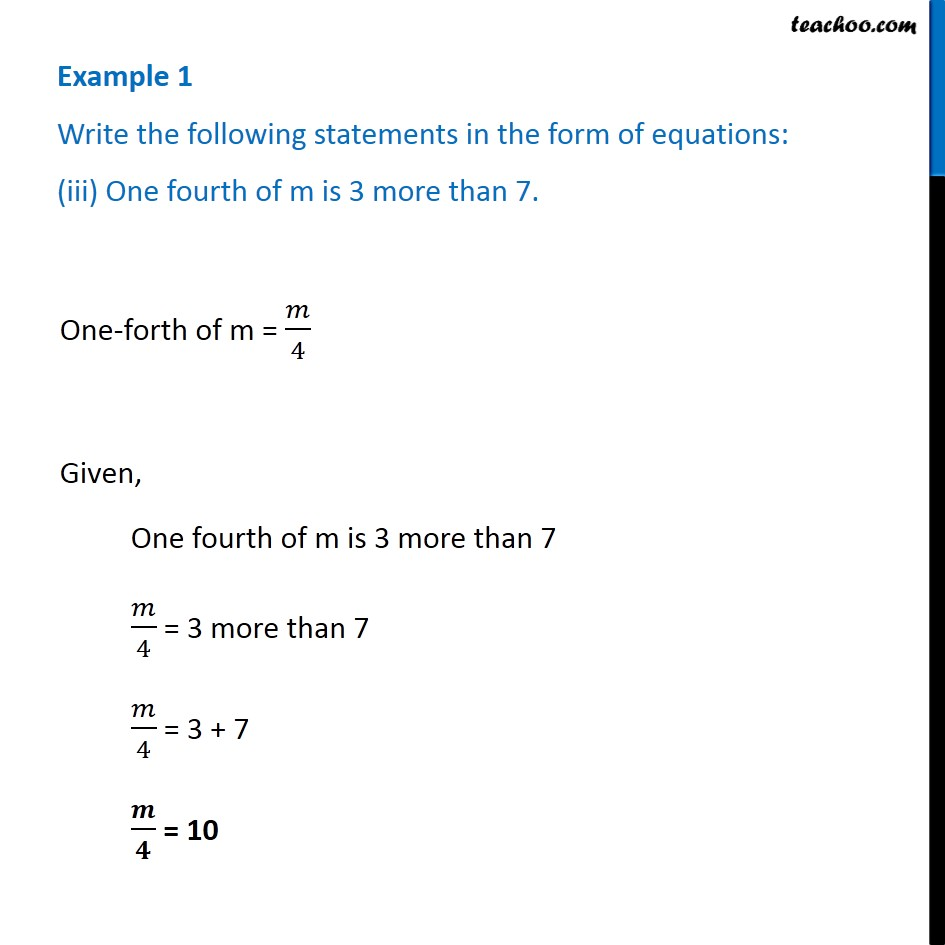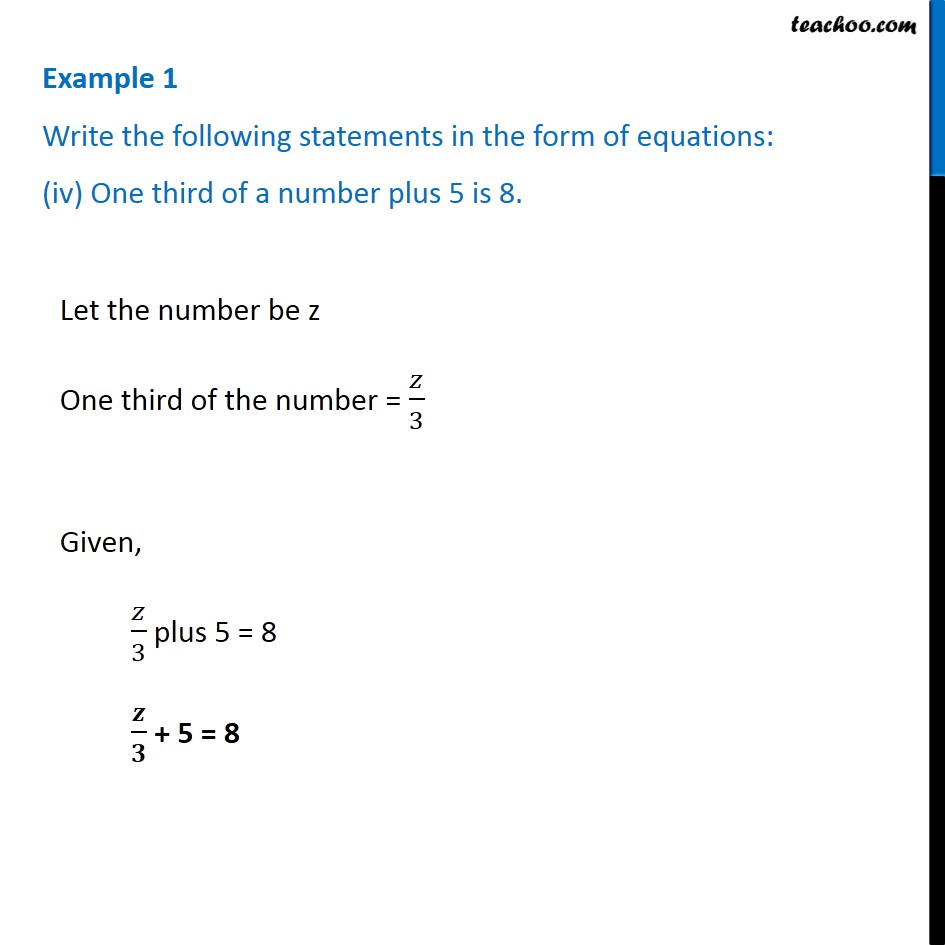Subscribe to our Youtube Channel - https://you.tube/teachoo

1. Chapter 4 Class 7 Simple Equations
2. Serial order wise
3. Examples

Transcript

Example 1 Write the following statements in the form of equations: (i) The sum of three times x and 11 is 32. 3 times x = 3x Given, Sum of 3x & 11 = 32 3x + 11 = 32 Example 1 Write the following statements in the form of equations: (ii) If you subtract 5 from 6 times a number, you get 7.Let the number be y 6 times a number = 6y Given, 6y minus 5 = 7 6y − 5 = 7 Example 1 Write the following statements in the form of equations: (iii) One fourth of m is 3 more than 7. One-forth of m = 𝑚/4 Given, One fourth of m is 3 more than 7 𝑚/4 = 3 more than 7 𝑚/4 = 3 + 7 𝒎/𝟒 = 10 Example 1 Write the following statements in the form of equations: (iv) One third of a number plus 5 is 8. Let the number be z One third of the number = 𝑧/3 Given, 𝑧/3 plus 5 = 8 𝒛/𝟑 + 5 = 8

Examples